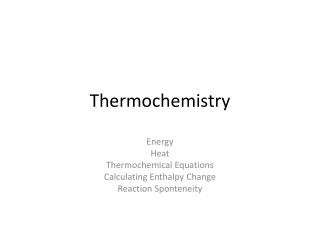DownloadDownload PresentationThermochemistry

# Thermochemistry

Download Presentation## Thermochemistry

- - - - - - - - - - - - - - - - - - - - - - - - - - - E N D - - - - - - - - - - - - - - - - - - - - - - - - - - -
##### Presentation Transcript

1. Thermochemistry Energy Heat Thermochemical Equations Calculating Enthalpy Change Reaction Sponteneity

2. The Nature of Energy All chemical reactions and changes in the physical state of substances involve either the release or absorption of heat. • Energy– the ability to do work or produce heat. • Chemical potential energy – energy stored in a substance because of its composition. • Heat – energy in the process of flowing from a warmer object to a cooler object. • Law of conservation of energy – in any chemical reaction or physical process, energy can be converted to one form to another, but it is neither created nor destroyed. • Thermochemistry– the study of heat changes that occur during chemical reactions and physical changes of state.

3. Heat Capacity • Calorie – the amount of energy required to raise the temperature of one gram of pure water by one degree Celsius. (1 Calorie(kCal) = 1000 cal) • Joule – SI unit of energy and of heat. (1 Joule = .2390 calories; 1 calorie = 4.184 joules) • Heat capacity – the amount of heat it takes to change an object’s temperature by exactly 10C.

4. Concept Practice These examples should go in your notes as we work them in class! (Pg. 265) • #3 • #4 • #5 • #6 • #7

5. Specific Heat Capacity • Specific heat – the amount of heat required to raise the temperature of one gram of that substance by one degree Celsius. Thermal Energy Equation: Q = m C T (examples worked in class) (Pg. 267)

6. Assignment • Pg. 286, 33 – 38 (due Monday (2/3))

7. Exothermic and Endothermic Processes • Surroundings – everything outside the system. • System – the specific part of the universe on which you focus your attention. • Example: A body is a system, what surrounds the body (environment) is the surroundings. • A fire warms you, an air conditioner cools you. • Universe – the system and surroundings together. • Endothermic process – process that absorbs heat from the surroundings. • Exothermicprocess – process that loses heat to the surroundings.

8. Calorimetry • Calorimeter – device used to measure the amount of heat absorbed or released during chemical reactions or physical processes. • Calorimetry – the accurate and precise measurement of the heat change for chemical and physical processes. • Enthalpy (H) - the heat content for systems at a constant pressure. • Heat and enthalpy are the same thing. - Q = H

9. Thermal Energy Equation • Q = m x c x T Q = heat (enthalpy) or( H) (J) m = mass (g) c = specific heat (J/g x 0 C) T = final temp. – initial temp. (0C)

10. Example 2 (pg. 271) • In-class assignment: • Pg. 268, 10 and 11 • Pg. 271, practice problem 15 • Due on Wednesday: • Pg. 287, 39-43